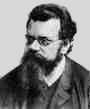Home

PHY 418: Statistical Mechanics I
Prof. S. Teitel stte@pas.rochester.edu ---- Spring 2012

## Problem Set 6

Due Monday, April 30, in lecture

• Problem 1 [15 points]

a) Find the chemical potential µ for an ideal, non-relativistic, three dimensional Fermi gas at low temperature, to second order in T, at fixed pressure p. Note, this is different from what we did in lecture - there we computed µ at fixed density N/V. (Hint: what is E/V at fixed p? You may wish to review Lecture 22 to do this problem.)

b) The Gibbs free energy is related to the chemical potential by G(T,p,N) = Nµ(T,p). The entropy can be derived from the Gibbs free energy, and hence from the chemical potential, by using,

S = - (dG/dT)p,N = - N(dµ/dT)p
Using your result from part (a), find the entropy of the Fermi gas to lowest order in T. Does your result agree with that given in Pathria §8.1 Eq.(41), as derived there from the Helmholtz free energy?

• Problem 2 [15 points]

Consider a non-interacting gas of spin zero bosons, whose energy-momentum relationship is given by ε(p) = A|p|s, for some fixed positive numbers A and s. The dimensionality of the gas is the number d, i.e. the volume of the gas is V = Ld, for a system of length L. In the following parts, we are considering the behavior in the thermodynamic limit of V → ∞.

a) For what values of s and d will there exist Bose-Einstein condensation at sufficiently low temperatures?

b) For the case that there is Bose-Einstein condensation, write an expression that gives how the condensate density no depends on the temperature T, the condensation temperature Tc, and the total density n.

c) Show that the pressure is related to the energy by, p = (s/d)(E/V)

d) Can a gas of photons in d = 3 undergo Bose-Einstein condensation?

• Problem 3 [15 points]

N Fermions A of spin 1/2 (i.e. gs=2) are introduced into a large volume V at temperature T. Two Fermions may combine to create a Boson with spin 0 (i.e. gs=1)via the interaction,

A + A ↔ A2
Creation of the molecule A2 costs energy εo > 0. At equilibrium, the system will contain NF Fermions and NB Bosons. Provide expressions from which the ratio NB/NF can be calculated, and perform the calculation explicitly for T=0. What would this (T=0) ratio be, if the particles were classical (i.e. quantum statistics could be neglected). Explain the difference.

• Problem 4 [10 points]

Consider the Ising model. The magnitization density is m, and the magnetic field is h. The magnetic susceptibility is then defined by

 χ = dmdh
where the derivative is evaluated at h = 0.

Derive an expression for χ in terms of the fluctuations in magnetization m. (Hint: recall, we had a similar expression for specific heat in terms of fluctuations in energy, and for compressibility in terms of fluctuations in volume.)